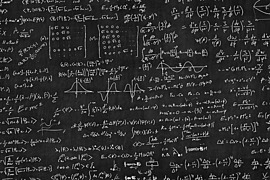## Terms# Mathematical Topics

Mathematics deals with logical reasoning and quantitative calculation. Adjunct to the physical sciences and technology since the 17th century, it has been the systematic treatment of magnitude, relationships between figures and forms, and relations between quantities. Some of the more used mathematical topics include Algebra, Geometry, Calculus, Logic, Number Theory, and Probability and Statistics.